# Faces2mp4: Faces to movie

A photographical timelapse project

This Jupyter notebook is for turning a series of selfies into a movie, by automatically finding-cropping and assembling each image provided. The time between each image is proportional of the time that passed between each picture, keeping the same rate.

Here is the final result:

This kind of utility most likely exists, but I wanted to build my own version that I could tune to my desire.

This script uses all the `.jpg` images in the current directory, having a known filename that contains date and time information.

## Get the source files

Let's start by getting and filtering all the images based on their extension:

``````import os

files = [file.name for file in os.scandir(".")
if os.path.splitext(file).upper() == ".JPG"
]
files.sort()
``````

# Perform the face detection

Let's have a batch opening and processing of the images, detecting all the faces one after the other. In case multiple faces are found for the first image, it uses the biggest face found. For the subsequent images, it is relying on the face that has the closest size to the one found in the first image.

``````import cv2
import numpy as np
import matplotlib.pyplot as plt
%matplotlib inline

# Helper function

def convertToRGB(image):
return cv2.cvtColor(image, cv2.COLOR_BGR2RGB)

# Load the face detection classifier (source: https://github.com/opencv/opencv/tree/master/data/)

faces = []
img_shapes = []
first_surface = 0
for img in files:
# Open source data
img_shapes.append(img_raw.shape)

# The face detection itself
minSizeFace = int(img_raw.shape/10)  # minimum size of a face
img_raw, minSize=(minSizeFace, minSizeFace))
print('Faces found in {} : {}'.format(img, len(faces_detected)))

# Compute the surface of the biggest face detected in the first image
# (it will be used later when multiple faces are detected, to select the closest match)
if first_surface == 0:
first_surface = max([w*h for (x, y, w, h) in faces_detected])

# We want not more than a face, and not fewer than a face. Let's find the best one
best = 0  # which face in the detection array is the best

if len(faces_detected) == 0:
# Create two faces detected from the previous image as an approximation raising a warning
# This assumes at least a face has been detected in the first image
faces_detected = []
faces_detected.append(faces[-1])
faces_detected.append(faces[-1])

if len(faces_detected) > 1:
# We have detected multiple faces, select the one closest in size to the one in the first image
plt.figure(num=None, figsize=(26, 6), dpi=80)
# Face highlight thickness depends on the image size
thickness = int(max(img_raw.shape)/100)

# Compute the face's surface difference from first image, to find the best one
surface_diffs = [abs(w*h - first_surface)
for (x, y, w, h) in faces_detected]
best = surface_diffs.index(min(surface_diffs))

for j, faces_rect in enumerate(faces_detected):
(x, y, w, h) = faces_rect  # unpack face rectangle properties

# Blur the face because of the Internetz
sub_face = img_raw[y:y+h, x:x+w]
sub_face = cv2.medianBlur(sub_face, int(w/6/2)*2 + 1)
img_raw[y:y+sub_face.shape, x:x+sub_face.shape] = sub_face

cv2.rectangle(img_raw, (x, y), (x+w, y+h),
(0, 255*(j == best), 255*(j != best)), thickness)

plt.imshow(convertToRGB(img_raw))
plt.title(img)

faces.append(faces_detected[best])
``````
``````Faces found in 20200314_210412.jpg : 1
…
Faces found in 20200422_210109.jpg : 1
Faces found in 20200423_210152.jpg : 2
Faces found in 20200424_211910.jpg : 1
…
Faces found in 20200602_000116.jpg : 1
Faces found in 20200602_205834.jpg : 0
Faces found in 20200603_210022.jpg : 1
…
Faces found in 20200622_230101.jpg : 1
``````

Now we have the face data, let's have a rough preview of what we have for the first few images:

``````plt.figure(num=None, figsize=(18, 6), dpi=60)
for i, (img, faces_rect, shape) in enumerate(zip(files, faces, img_shapes), 1):
# Preview for the first 5 images
if i > 5:
continue

# Prepare the display
plt.subplot(1, 5, i)
plt.title(img)

# Get/compute parameters
# Face highlight thickness depends on the image size
thickness = int(max(img_raw.shape)/100)
(x, y, w, h) = faces_rect  # unpack face rectangle properties

# Blur the face because of the Internetz
sub_face = img_raw[y:y+h, x:x+w]
sub_face = cv2.medianBlur(sub_face, int(w/4/2)*2 + 1)
img_raw[y:y+sub_face.shape, x:x+sub_face.shape] = sub_face

# Highlight the face
cv2.rectangle(img_raw, (x, y), (x+w, y+h), (0, 255, 0), thickness)

plt.imshow(convertToRGB(img_raw))
``````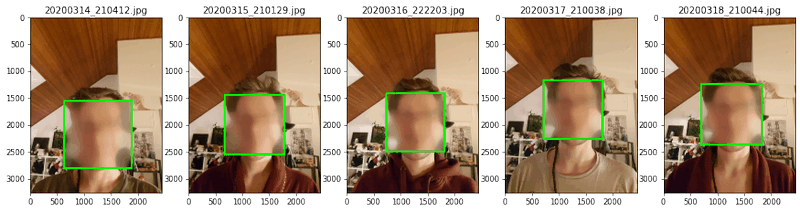# Scale, crop, and save the images

Now, we can scale and crop all the images around the face, giving little margins everywhere. The face is modeled from the average detected aspect-ratio.

``````ratio = 0.
for face_rect in faces:
(x, y, w, h) = face_rect
ratio += float(w)/float(h)

ratio /= len(faces)

assert int(ratio * 100) == 100
``````

It looks like the classifier returns not any kind of rectangle, but exactly a square (with at least two decimals). Or my face is super square, which is also possible, but I wouldn't have guessed. Let's scale all the images so that all faces have the same size, canceling (some) problems of being closer or further than the camera.

``````import math

smallest = math.inf

for x, y, w, h in faces:
smallest = min(smallest, w)

scalings = []
scaled_shapes = []
scaled_faces = []
for (H, W, _), (x, y, w, h) in zip(img_shapes, faces):
s = smallest/w
s /= 1.3  # My images are big, I can scale them further
scalings.append(s)

scaled_shapes.append((int(s*H), int(s*W)))
scaled_faces.append((int(x*s), int(y*s), int(w*s), int(h*s)))
``````

Let's get what can be the biggest margins for our images

``````from functools import reduce

margins = {}
for (H, W), (x, y, w, h) in zip(scaled_shapes, scaled_faces):
margins['top'] = int(math.floor(min(margins.get('top', math.inf), y)))
margins['left'] = int(math.floor(min(margins.get('left', math.inf), x)))
margins['bottom'] = int(math.floor(
min(margins.get('bottom', math.inf), H-(y+h))))
margins['right'] = int(math.floor(
min(margins.get('right', math.inf), W-(x+w))))
print("Margins:", margins)
``````
``````Margins: {'top': 137, 'left': 70, 'bottom': 75, 'right': 119}
``````

Now, we can resize and crop all the images to the same canvas.

``````plt.figure(num=None, figsize=(18, 6), dpi=60)
imgs = []
cropped_faces = []
for i, (path, (x, y, w, h), s) in enumerate(zip(files, scaled_faces, scalings), 1):

# Resize image
img = cv2.resize(img, (0, 0), fx=s, fy=s)

# Crop image, being encoder friendly with cropping being multiple of 2
if (margins["top"]+h+margins["bottom"]) % 2 != 0:
margins["top"] = margins["top"] - 1
if (margins["left"]+w+margins["right"]) % 2 != 0:
margins["right"] = margins["right"] - 1
crop_img = img[y-margins["top"]:y+h+margins["bottom"],
x-margins["left"]:x+w+margins["right"]]
crop_face = (margins["left"], margins["top"], w, h)

# Save face position in the cropped images
cropped_faces.append(crop_face)
imgs.append(crop_img.copy())

# Blur face for online publication
(x, y, w, h) = crop_face
sub_face = crop_img[y:y+h, x:x+w]
sub_face = cv2.medianBlur(sub_face, int(w/4/2)*2 + 1)
crop_img[y:y+sub_face.shape, x:x+sub_face.shape] = sub_face

# Save image (disabled as we'll export the morphing images)
# cv2.imwrite('image_{}.png'.format(i),crop_img)

# Preview for the first 5 images
if i > 5:
continue

# Blur the face because of the Internetz
(x, y, w, h) = crop_face
sub_face = crop_img[y:y+h, x:x+w]
sub_face = cv2.medianBlur(sub_face, int(w/4/2)*2 + 1)
crop_img[y:y+sub_face.shape, x:x+sub_face.shape] = sub_face

plt.subplot(1, 5, i)
plt.title(" ".join([path, "crop"]))
plt.imshow(convertToRGB(crop_img))
``````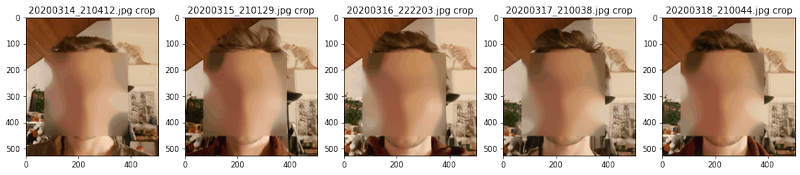# Time information

As an added bonus, it would be better to take into account the photo capture time when assembling the video. We therefore first need to parse the date-time from the image filenames, and I will normalize it between 0 and 1.

``````from datetime import datetime

plt.figure(num=None, figsize=(18, 6), dpi=60)

total_time = 30  # seconds of the video
fps = 25

# Parse
date_times = [datetime.strptime(file, '%Y%m%d_%H%M%S.jpg') for file in files]

# Zero-offset
date_times = [dt - date_times for dt in date_times]

# Normalize from 0 to 1
date_times = [dt/date_times[-1] for dt in date_times]

# Stretch to `total_time` seconds seconds
date_times = [dt*total_time for dt in date_times]

# For each interval, for each frame, get the progression
progressions = []
for i, (t1, t2) in enumerate(zip(date_times[0:-1], date_times[1:]), 1):
delta = t2-t1
num_img = math.ceil(delta*fps)
progress = np.linspace(0, 1, num_img, endpoint=False)

# Ease in and out
power = 2.5  # Defines how sharp the transition is
progress_ease = progress**power / (progress**power + (1 - progress)**power)

progressions.append(progress_ease)

if i > 5:
continue

plt.subplot(1, 5, i)
plt.plot(progress, progress_ease, '.')
plt.title("Progress image {} → {}".format(i, i+1))
``````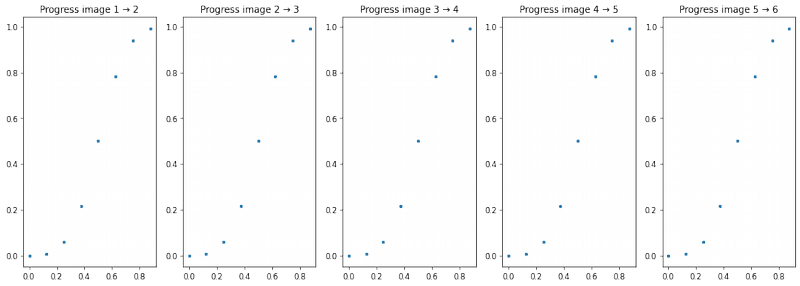# Face landmarks for morphing interpolation

Yep, this title is full of keywords. Except it's maybe missing "deep learning", but it's because there is none here. I'm just using off-the-shelf tools and pre-trained models.

## Landmark identification

We first get the specific points of the face:

``````import numpy

plt.figure(num=None, figsize=(18, 6), dpi=60)

facemark = cv2.face.createFacemarkLBF()
# https://github.com/kurnianggoro/GSOC2017/blob/master/data/lbfmodel.yaml

mark_points = []
for i, (img, face) in enumerate(zip(imgs, cropped_faces), 1):
ok, landmarks = facemark.fit(img, numpy.array([face]))

# Get all the points out of their structure
points = []
for mark in landmarks:
for sub in mark:
for point in sub:
points.append(point)
mark_points.append(points)

# Preview for the first 5 images
img = img.copy()
if i > 5:
continue

# Blur the face because of the Internetz
(x, y, w, h) = face
sub_face = img[y:y+h, x:x+w]
sub_face = cv2.medianBlur(sub_face, int(w/4/2)*2 + 1)
img[y:y+sub_face.shape, x:x+sub_face.shape] = sub_face

for (x1, y1) in points:
r = int(img.shape/200)
color=(255, 0, 0), thickness=int(r/2))

plt.subplot(1, 5, i)
plt.title(" ".join([path, "marks"]))

plt.imshow(convertToRGB(img))
``````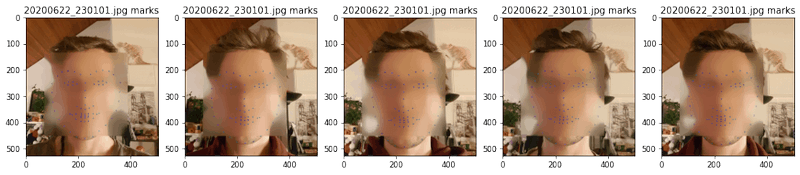## Triangulation

We can create now a map of triangles with the Delaunay algorithm. We will create a few additional points of the border of the image to bind the morphing to the sides.

``````subdivs = []
triangles = []
for img, mark in zip(imgs, mark_points):
# Create points on the border
for i in range(3):
for j in range(3):
if i == 1 and j == 1:
continue
point = (float(i)*(img.shape-1)/2, float(j)*(img.shape-1)/2)
mark.append(point)

# Rectangle to be used with Subdiv2D
size = img.shape
rect = (0, 0, size, size)
subdiv = cv2.Subdiv2D(rect)

for (x, y) in mark:
# We can't add points as floats, need to be ints
subdiv.insert((int(x), int(y)))

subdivs.append(subdiv)
triangles.append(subdiv.getTriangleList())
``````

Let's have a view over the delightful triangulation:

``````plt.figure(num=None, figsize=(18, 6), dpi=60)
for i, (img, points, subdiv, tris) in enumerate(zip(imgs, mark_points, subdivs, triangles), 1):
# Preview for the first 5 images
img = img.copy()
if i > 5:
continue

# Blur the face because of the Internetz
(x, y, w, h) = face
sub_face = img[y:y+h, x:x+w]
sub_face = cv2.medianBlur(sub_face, int(w/4/2)*2 + 1)
img[y:y+sub_face.shape, x:x+sub_face.shape] = sub_face

# Draw Delaunay triangulation
size = img.shape
r = (0, 0, size, size)
color = (255, 255, 0)

for t in tris:
pt1 = (t, t)
pt2 = (t, t)
pt3 = (t, t)
cv2.line(img, pt1, pt2, color)
cv2.line(img, pt2, pt3, color)
cv2.line(img, pt3, pt1, color)

for (x1, y1) in points:
r = int(img.shape/200)
color=(255, 0, 0), thickness=int(r/2))

plt.subplot(1, 5, i)
plt.title(" ".join([path, "crop"]))

plt.imshow(convertToRGB(img))
``````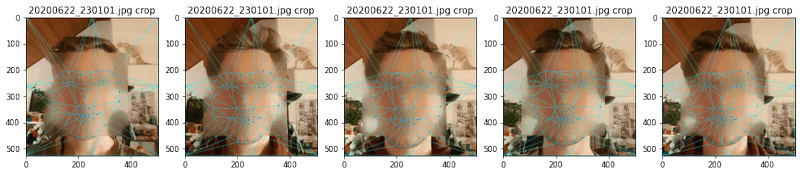# Morphing

Code courtesy from LearnOpenCV, with many tweaks to get it to work with what I have. We start with some definitions, then actually using the functions.

``````# Apply affine transform calculated using srcTri and dstTri to src and
# output an image of size.
def applyAffineTransform(src, srcTri, dstTri, size):

# Given a pair of triangles, find the affine transform.
warpMat = cv2.getAffineTransform(np.float32(srcTri), np.float32(dstTri))

# Apply the Affine Transform just found to the src image
dst = cv2.warpAffine(src, warpMat, (size, size), None,
flags=cv2.INTER_LINEAR, borderMode=cv2.BORDER_REFLECT_101)

return dst

# Warps and alpha blends triangular regions from img1 and img2 to img
def morphTriangle(img1, img2, img, t1, t2, t, alpha):

# Find bounding rectangle for each triangle
r1 = cv2.boundingRect(np.float32([t1]))
r2 = cv2.boundingRect(np.float32([t2]))
r = cv2.boundingRect(np.float32([t]))

# Offset points by left top corner of the respective rectangles
t1Rect = []
t2Rect = []
tRect = []

for i in range(0, 3):
tRect.append(((t[i] - r), (t[i] - r)))
t1Rect.append(((t1[i] - r1), (t1[i] - r1)))
t2Rect.append(((t2[i] - r2), (t2[i] - r2)))

# Get mask by filling triangle
mask = np.zeros((r, r, 3), dtype=np.float32)
cv2.fillConvexPoly(mask, np.int32(tRect), (1.0, 1.0, 1.0), 16, 0)

# Apply warpImage to small rectangular patches
img1Rect = img1[r1:r1 + r1, r1:r1 + r1]
img2Rect = img2[r2:r2 + r2, r2:r2 + r2]

size = (r, r)
warpImage1 = applyAffineTransform(img1Rect, t1Rect, tRect, size)
warpImage2 = applyAffineTransform(img2Rect, t2Rect, tRect, size)

# Alpha blend rectangular patches
imgRect = (1.0 - alpha) * warpImage1 + alpha * warpImage2

# Copy triangular region of the rectangular patch to the output image
img[r:r+r, r:r+r] = img[r:r + r,
``````

We can now use the defined functions to perform the morphing.

``````current_image = 0
for (img1, points1, subdiv1, tris1, img2, points2, subdiv2, tris2, alphas, face) in zip(imgs[0:-1], mark_points[0:-1], subdivs[0:-1], triangles[0:-1], imgs[1:], mark_points[1:], subdivs[1:], triangles[1:], progressions, cropped_faces):
for alpha in alphas:
# Allocate space for final output
points = []
imgMorph = alpha * img1 + (1-alpha) * img2

# Compute the intermediate points position
for i in range(0, len(points1)):
x = (1 - alpha)*points1[i] + alpha*points2[i]
y = (1 - alpha)*points1[i] + alpha*points2[i]
points.append((x, y))

# Create the triangles from the list of points (to be sure we have the same ordering)
triangle_ids = []
for t1 in tris1:
distances = np.apply_along_axis(np.linalg.norm, 1,
np.round(abs(np.subtract(points1, np.array([t1, t1])))))
i1 = np.where(distances == np.amin(distances))
distances = np.apply_along_axis(np.linalg.norm, 1,
np.round(abs(np.subtract(points1, np.array([t1, t1])))))
i2 = np.where(distances == np.amin(distances))
distances = np.apply_along_axis(np.linalg.norm, 1,
np.round(abs(np.subtract(points1, np.array([t1, t1])))))
i3 = np.where(distances == np.amin(distances))
triangle_ids.append([i1, i2, i3])

t1 = []
t2 = []
t = []
for (a, b, c) in triangle_ids:
x = int(a)
y = int(b)
z = int(c)

t1 = [points1[x], points1[y], points1[z]]
t2 = [points2[x], points2[y], points2[z]]
t = [points[x], points[y], points[z]]

# Morph one triangle at a time.
morphTriangle(img1, img2, imgMorph, t1, t2, t, alpha)

# Blur the face because of the Internetz
#(x,y,w,h) = face
#sub_face = imgMorph[y:y+h, x:x+w]
#sub_face = cv2.medianBlur(sub_face, int(w/4/2)*2 + 1)
#imgMorph[y:y+sub_face.shape, x:x+sub_face.shape] = sub_face

# Save images
cv2.imwrite('image_morph_{}.png'.format(current_image), imgMorph)
current_image += 1
``````

# Merging to a video

We now have a ton of `.png` images, let's turn that into a video. Even though there is a `ffmpeg` python wrapper, I do not think it is pretty useful for such a simple usage:

``````ffmpeg -r 25 -f image2 -i image_morph_%d.png -vcodec libx264 -preset veryslow -crf 21 -profile:v baseline -level 3.0 -pix_fmt yuv420p beard_morph.mp4
``````

This command sets all the right options of a well compressed and widely compatible file that can be played on most smartphones.